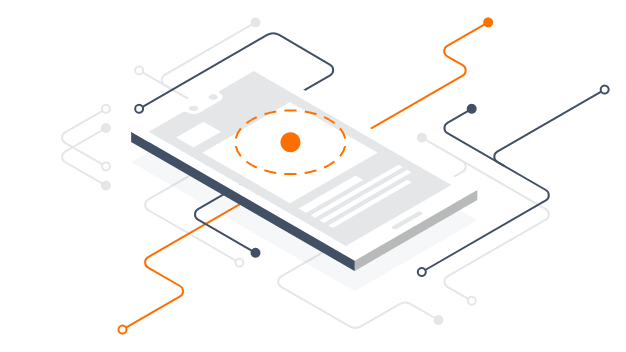# Optimization techniques – TFLite!!

Source: Deep Learning on Medium## What is Quantization?

Quantization is generally used in mathematics and digital signal processing. Below is the wiki definition.

Quantization, in mathematics and digital signal processing, is the process of mapping input values from a large set (often a continuous set) to output values in a (countable) smaller set, often with a finite number of elements. Rounding and truncation are typical examples of quantization processes.

Quantization refers to the process of reducing the number of bits that represent a number. In the context of deep learning, the dominant numerical format used for research and for deployment has so far been a 32-bit floating-point or FP32. Convert FP32 weights and output activations into the nearest 8-bit integer, some times 4/2/1 bit as well in quantization.

Quantization optimizes the model by quantizing the weights and activation type. TFLite uses quantization technique to speed up inference over the edge devices. TFLite converter is the answer to whether we can manage a deep learning model with lower precision. Now you know exactly quantization, let us, deep dive:

Quantization dramatically reduces both the memory requirement and computational cost of using neural networks.

The quantizing deep learning model uses techniques that allow for reduced precision representations of weights and, optionally, activations for both storage and computation.

TFLite provides several level of support to quantization.

1. Post-training quantization
2. Quantization aware training.

Below is a table that shows the benefits of model quantization for some CNN models.

## Post-training quantization:

As the name implies its post-training technique, this is after your model is trained. Post-training quantization is a technique used to quantizing weights and activation types. This technique can reduce the model size and also improving CPU and hardware acceleration latency. There are different optimization options such as weight, full integer, etc based on our requirement we can choose.